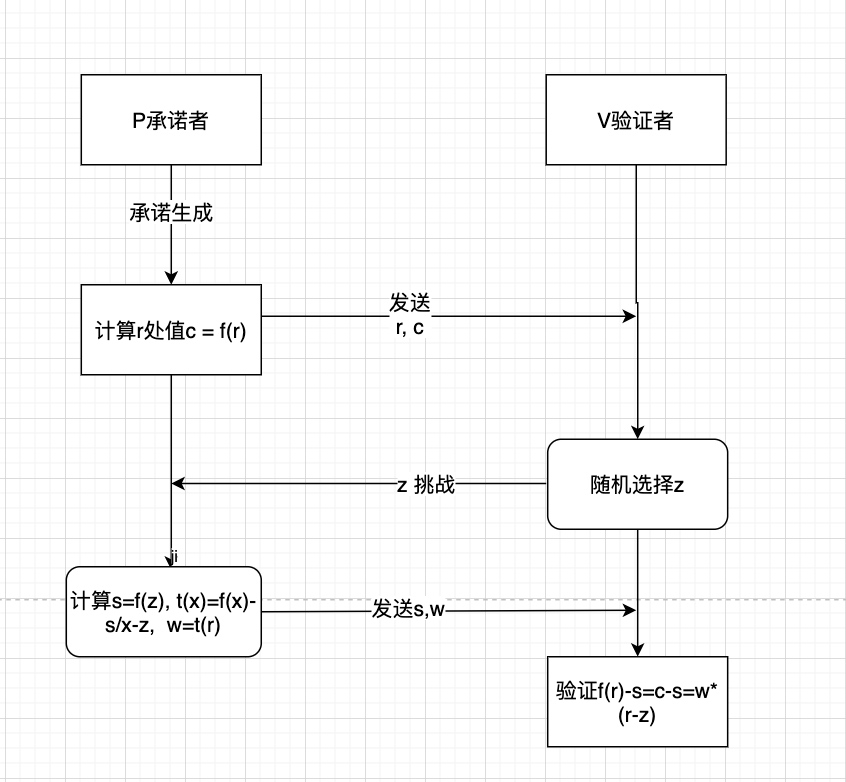## 何为多项式

### 多项式

\$f(x)=a_0 + a_1x + ... + a_nx^n ={a_0,a_1,...,a_n}\$

\$f(x)={(x_0,y_0),(x_1,y_1), ... ,(x_n,y_n)}\$

## 多项式承诺

### 点值承诺

1. 承诺生成（Commit）阶段： 承诺方选择一个暂不公开的多项式，在某一点r处，计算出对应的承诺c并公开。c = f(r), 将（r，c）公开给验证方
2. 承诺披露（Reveal）阶段： 承诺方公布多项式，验证方根据多项式计算r处值c' = f(r)，比较c'= c，一致则表示验证成功，否则失败。

1. 承诺生成（Commit）阶段： 承诺方选择一个暂不公开的多项式，在某一点r处，计算出对应的承诺c并公开。c = f(r), 将（r，c）公开给验证方
2. 挑战（challenge）与证明生成： 验证方V随机选择一个数z,发给承诺方P, P计算在z处值s = f(z)，同时计算出t(x) = f(x)-s / (x-z),计算t(x)在z处的值w = t(z)(w也称为见证witness) 返回给验证方V(s,w)
3. 验证阶段： 验证方验证：s = f(z) --> f(z) - s = 0 --> 方程f(x)-s = 0 有根x=z, 即存在t(x) 使得f(x) - s = t(x)(x - z), 这个方程是恒等式，所以任意点都成立。 在r处自然也是成立的，所以可以检验f(r) - s = t(r)(r - z) = c - s = w(r - z ) 通过则验证成功，否则失败。## 小结

### 相关阅读

Schorr签名与椭圆曲线 Schorr签名与椭圆曲线

• 发表于 2021-02-22 17:35
• 阅读 ( 2520 )
• 学分 ( 5 )
• 分类：入门/理论